# Graph of a mappingof a setinto a setThe subsetof the product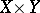consisting of the points,. Ifandare topological spaces,is a continuous mapping andis the projection of the topological productonto the factor, then the mappingis a homeomorphism of the subspaceonto. Ifis a Hausdorff space, then the setis closed in the product.

B.A. Pasynkov

In the case of a real-valued functionofreal argumentsand domain of definition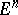, its graph is the set of all ordered pairs, whereis any point of; in other words, it is the set of all points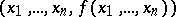in. Having chosen a coordinate system (Cartesian, polar or any other coordinates), the numerical points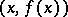,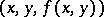can be represented by points of the plane or space. For real-valued functionsin one real variable which have derivatives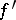,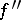, in more or less complicated examples the graph can be sketched by studying the signs ofand. The sign ofis an indicator of the monotony of, while the sign ofindicates the direction of convexity of the graph of the function. To obtain an idea on the graph of a real-valued functionin two real variables, the method of sections may be employed: One studies the sections of the graph by certain planes, in particular by planes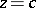; the projection of this section on the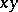-plane is said to be a level set of. Similarly, for a functiondefined in a domain, the level set ofat level, whereis an arbitrary number, is the set of all solutions of the equation. The solutions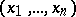must be found in. A level set may prove to be empty. If a level set is a line or a surface, it is known as a level line or a level surface of the function.

A.A. Konyushkov

An extremely important theorem in functional analysis is the so-called closed-graph theorem: Ifandare Fréchet spaces (cf. Fréchet space) andis a linear mapping with a closed graph, thenis continuous. Many generalizations of this result are known (see [a1]).